## Annotation

When performing mathematical operations with infinite series, it is necessary to follow the rules of the positional number system.

## Number of terms

The number of terms of an infinite series, presented in the visible part of the series, must be considered as an analogue of writing a number using numbers in the positional number system. The number of terms in the visible part of the series is similar to the rounding of an ordinary decimal fraction with a certain accuracy, that is, to a certain number of decimal places.

When performing mathematical operations with infinite series, for each example it is necessary to use the same number of terms in the visible part of the series. If you do not follow this rule, it can lead to an erroneous result. In mathematics, it is not customary to use any number with different rounding accuracy in one example.

## Shifting series

Shifting an infinite series when performing mathematical operations automatically leads to an incorrect result. This is analogous to adding zeros to the decimal immediately after the decimal point.

Consider an example. If you subtract the same series without a shift from any series, the result will be zero. If the subtraction uses a shift and does not take into account the compensating group of terms, then the result will be different from zero. Suppose we have a series S. Let's write the series -S and add these two series. The result should be zero. By performing addition without shift, we get the correct result.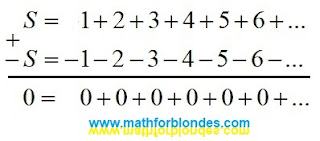Addition of infinite series without shift

Shift by one position leads to wrong result.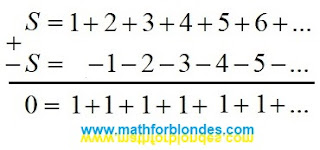Shift by one position

A two-position shift leads to another incorrect result.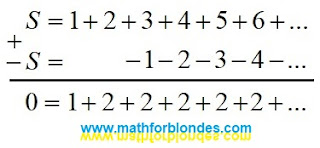A two-position shift

Shifts by an arbitrary number of positions allow you to get an infinite number of incorrect results.

Compliance with the rule about the same number of terms of an infinite series in one example and the use of the compensating group of terms (highlighted in curly brackets) avoids an error. But in the examples given, this method is more laborious than no shift.The use of a compensating group of terms

Let us analyze some of the most well-known examples of determining the sums of infinite divergent series.

## Sum of Grandi's series

In the given example, the sum of Grandi's series is equal to 1/2, which is not a correct result.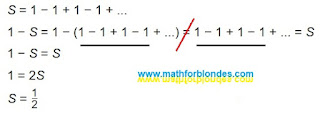Sum of Grandi's series

The second line contains an equals sign between two different sums: one sum consists of five terms that are not equal to zero, the second consists of four. In this example, the optical illusion of the equality of different sums is used, if one term is hidden behind the three-dots of infinity.

## The sum of the alternating series

Below is an alternating series and a solution for determining its sum.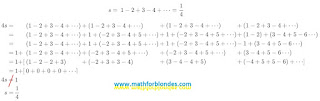The sum of the alternating series

In the proposed solution, the same series is represented by a different number of terms: from four to six. When performing mathematical operations and rearranging terms, the compensating group of terms is ignored. Two other methods for obtaining the sum 4s are not considered: addition of series without shift and multiplication of the original series s by 4. Both these methods give the same result, which indicates the mathematical accuracy of the calculations performed.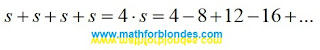The sum 4s

## Sum of natural numbers

Consider the sum of an infinite series of natural numbers. Intuitively, this is a divergent infinite series that cannot have a finite sum value. But, here is an example of calculating the sum of this infinite series.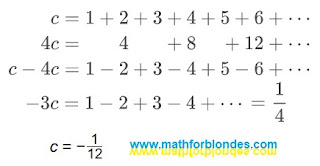Sum of natural numbers

Typical errors of these calculations are shown in the example above. Mathematicians did not check the solution: the original series c, multiplied by minus three, is equal to: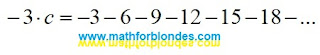Solution verification

## Conclusion

All the above ways of finding the sum of an infinite series are nothing more than fitting the solution to a given result. Nobody prevents mathematicians from establishing their own rules for virtual games in numbers. But the application of such results of "calculations" in the laws of physics leads to their misunderstanding. For example, a numerical coefficient due to some physical parameter or simply a correction factor is interpreted as the sum of some infinite series. An example of an erroneous interpretation of numerical coefficients would be the following statement: the area of ​​a right triangle is equal to the sum of the Grandi series multiplied by the product of its legs.